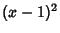## Degenerate

A limiting case in which a class of object changes its nature so as to belong to another, usually simpler, class. For example, the Point is a degenerate case of the Circle as the Radius approaches 0, and the Circle is a degenerate form of an Ellipse as the Eccentricity approaches 0. Another example is the two identical Roots of the second-order Polynomial. Since theRoots of anth degree Polynomial are usually distinct, Roots which coincide are said to be degenerate. Degenerate cases often require special treatment in numerical and analytical solutions. For example, a simple search for both Roots of the above equation would find only a single one: 1.

The word degenerate also has several very specific and technical meanings in different branches of mathematics.

References

Arfken, G. Mathematical Methods for Physicists, 3rd ed. Orlando, FL: Academic Press, pp. 513-514, 1985.